# How to Subtract in Google Sheets

Experienced Google Sheet users with an Excel background can efficiently perform complex mathematical operations using the free G-suite program. That’s because there’s a great similarity in the way calculations are performed in both Excel and Google Sheets.However, due to a few subtle differences, first-time users of Google Sheets might be baffled when trying to perform even the most basic functions, such as subtracting. If you belong to the second category, this article may be your Google Sheets lottery-winning ticket. You can walk away today richer in the knowledge of how to subtract all types of numerical data in Google Sheets.

## How to Subtract in Google Sheets

Google Sheets is a great program for working with numerical information. You can perform all sorts of calculations here, including subtraction. In fact, thanks to Google Sheets, you can perform hundreds of subtractions in one go. And to help you do your math correctly, Sheets lets you use Formulas and Functions, pretty much in the same way as Excel.

Let’s start by explaining how to subtract numbers in Sheets the most straightforward way – using a formula.

## How to Subtract in Google Sheets With a Formula

One of many time-saving features Google Sheets offers is the ability to subtract numbers. This G-suite app uses formulas, which are simply mathematical expressions to make calculations easier. Remember using formulas such as “10-5=5” in Grade 1? You can use this same expression to perform numerical calculations within the program. For subtracting, simply use a minus sign (-) in the formula as a mathematical operator.

One key difference from the common formulas you’re used to seeing (5-4=1) is that the equal sign in Sheets comes first. For detailed instructions on creating a subtraction formula, follow the steps below:

1. Open the Google Sheet where you need to subtract numerical information.
2. Select a cell where you want the total to appear.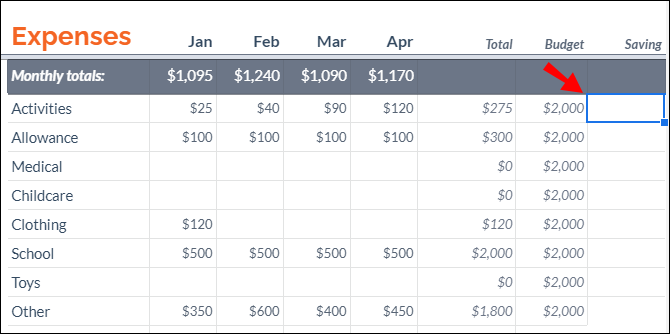3. Type the equal sign (=) in that cell.4. Insert cell references of the numerical information you need to subtract. For example, if you were to subtract the numerical data from the cells A5 and A6, you’d write “`=A5-A6`.”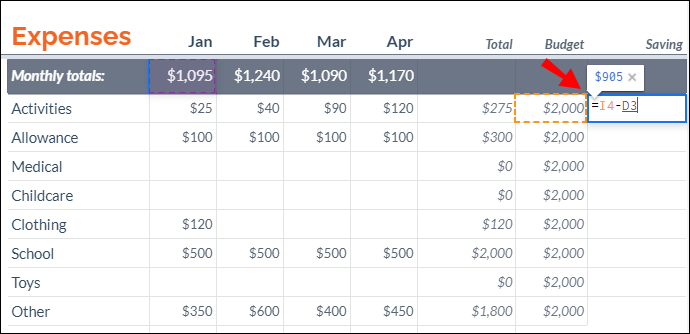5. Press the “Enter” or “Return” key on the keyboard to run the formula to subtract the numbers.You can also subtract cells without having to insert cell references (as shown in Step 4) manually. Simply apply the steps below:

1. Start a formula by typing an equal sign in a cell.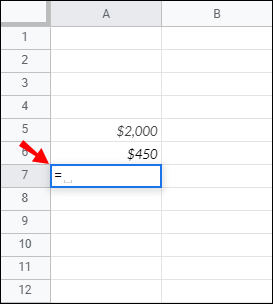2. Click on a cell you want to reference. In this case, it would be the cell with the value you wish to subtract: A5.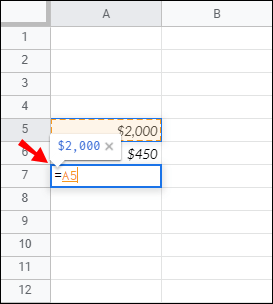4. Click on the second cell you want to reference. In this case, it’s the cell with the value you’re subtracting from the first cell: A6.Note: If you change the value of any cell reference, the total will recalculate automatically.

## How to Subtract Time in Google Sheets

To subtract time, you can either use basic math calculations or functions. However, with the latter, you can only subtract units less than 24 hours, 60 minutes, or 60 seconds.

### Subtracting Hours

You can use the following function to subtract less than 24 hours:

`[cell reference]-TIME(N hours,0,0).`

Let’s see how this would work on real data. If you were to subtract 4 hours from the cell B3, you would write the following formula:

`B3-TIME(4,0,0)`

To subtract more than 24 hours, use this formula:

`[cell reference]-(N hours/24)`

Let’s put this into practice. If you were to subtract 35 hours from the cell C2, you’d use this formula:

`C2-(35/24)`

### Subtracting Minutes

We use the same procedure to subtract minutes.

To subtract less than 60 minutes:

`[cell reference]-(0, N minutes, 0)`. For example: `B1-(0,30,0)`.

To subtract more than 60 minutes:

`[cell reference]-(N minutes/1440)`. For example: `B1-(90/1440)`.

### Subtracting Seconds

To subtract less than 60 seconds:

`[cell reference]-(0,0,N seconds)`. For example: `A4-(0,0,45)`

To subtract more than 60 seconds:

`[cell reference]-(N seconds/86400)`. For example: `A5-(124/86400)`.

## How to Subtract Dates in Google Sheets

The easiest way to calculate the difference in the number of days between two dates is to simply subtract them. We’ll use the same formula as with any other numerical information (e.g. C2-B2).

However, when subtracting the dates, Google Sheets doesn’t count the start date. For example, if you were to subtract days from May 10 (e.g., cell C2) until May 3 (e.g. cell B2), the total would include dates starting from May 4 up until May 10. If you want the calculation to include May 3, you’d have to add “+1” to the end of the formula (e.g. C2-B2+1)

## How to Subtract Columns in Google Sheets

Once you learned how to subtract cells with a formula, subtracting columns is a breeze. The easiest way to do so is to drag the fill handle from the cell you’ve applied the formula down to the last row of that column.

Here are the detailed instructions:

1. Type an equal sign in a cell where you want the total to appear.2. Click on a cell you wish to reference. In this case, it would be the cell that contains the value you wish to subtract.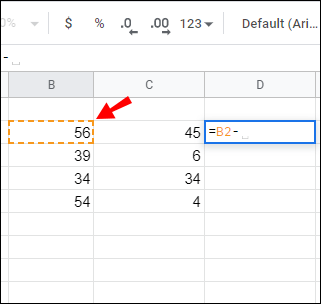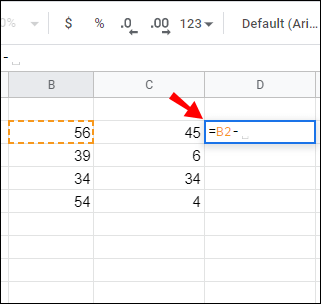4. Click on the second cell you wish to reference. In this instance, it’s the cell that contains the value you’re subtracting from the first cell.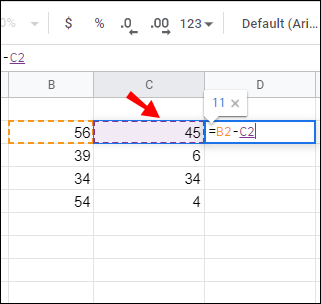5. When you get the first formula, it’s relatively easy to subtract that column’s remaining cells. Just hover the cursor over the cell showing the result of the first subtraction.6. Double-click on the square once your cursor changes into a plus sign. The formula will copy to all cells from that column. Alternatively, drag the fill handle down to the last row.## How to Subtract Percentage in Google Sheets

If you’re familiar with subtracting a percentage from a number in Excel, you can apply the same steps in Google Sheets. The formula is as follows: “=Total-Total*Percentage.”

Let’s say you have a value of 100 in cell C4. To subtract 20% from 100, apply the following steps:

1. Click on the cell where you want the total to appear (e.g. C5).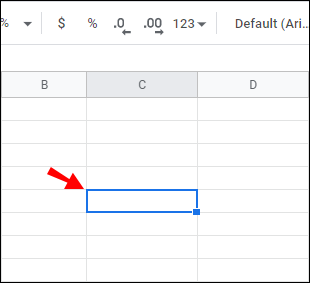2. Type the equal sign in that cell (e.g. C5) to initiate the formula.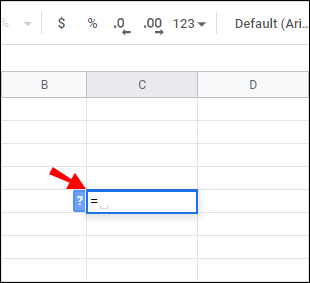3. Click on C4 to insert it as a cell reference.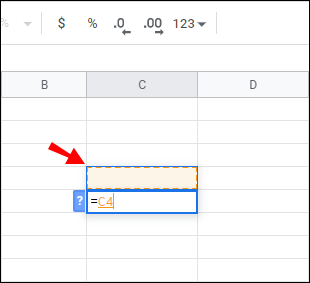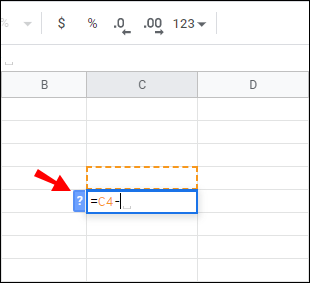5. Click on C4 again and type “`*” followed by “20%.`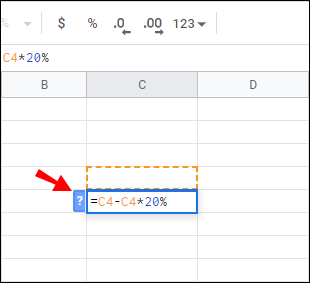6. This is how the complete formula should look in C5: “`=C4-C4*20%`.”## How to Subtract Function in Google Sheets

One feature that’s exclusive to Google Sheets is the MINUS function. Its syntax is “`MINUS(value1, value2)`,” and you can use it to subtract cell values. At this point, the MINUS function can only subtract two values, not more.

1. Type “`300`” in cell A3.2. Type “`200`” in cell A4.3. Select cell A5 and enter the following syntax: “`=MINUS(A3, A4)`” in the function bar.4. Cell A5 will return a value of 100 after you hit “Enter.”As easy as the MINUS function is to use, you might be better off subtracting numerical information with formulas due to its limited range (it subtracts only two cells at a time).

Here are some more questions you may have regarding subtracting in Google Sheets.

### How Do I Create Formula in Google Sheets?

Just as in Excel, Google Sheets uses standard formula operators:

• Subtraction: – (minus sign).

• Multiplication: * (asterisk).

• Division: / (forward slash)

• Exponents: ^ (caret)

You need to start each formula with an equal sign. Then, include two cell references that are parts of the mathematical operation, with a formula operator between them.

### How to Use the Sum Function in Google Sheets?

Google Sheets has the SUM function built in to help you add up columns or rows. Here’s what your SUM function should look like: “`=sum(CellA1, CellA2….CellA50)`.”

Follow the steps below for detailed instructions on how to use the SUM function:

• Click on a specific cell to apply the formula.• Start the formula by typing “`=sum(`“ and select the cells you wish to sum the values.• Type a closing parenthesis “`)`” and hit “Enter” to finish.• The sum of all the cells you selected will appear in the cell in which you previously started the function.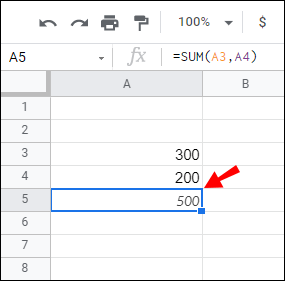To add columns, you can use the SUM function (see the paragraph above). Just follow the steps as explained.

You can either select all cells from the specific column manually (Step 2) or enter the cell range you wish to add. For example, if your goal is to calculate the sum of column A spreading from A1 to A5, you’d use the following formula: “`=sum(A1:A5)`” instead of “`=sum(A1,A2,A3,A4, A5)`.”

## Mastering Subtraction in Google Sheets

If you were puzzled about the idea of subtracting data in Google Sheets before reading this article, then hopefully this information has solved the mystery. Performing this operation merely includes two mathematical operators (equal and minus signs) and cell references. Whether your goal is to subtract time, date, percentage, or simple numbers, these tips should have you covered.

Finally, if you’re completely new to Google Sheets, remember that the equal sign always goes first, no matter what formula you apply.

What kind of data do you usually subtract in Google Sheets? Share your experiences in the comments section below.

Disclaimer: Some pages on this site may include an affiliate link. This does not effect our editorial in any way.

Todays Highlights